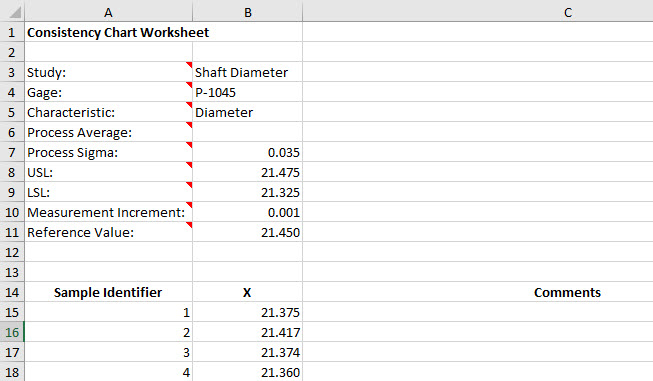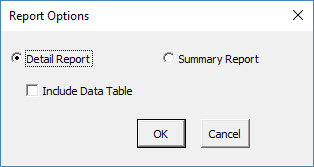# EMP Consistency Study HelpAn EMP consistency study is used to determine if the measurement system is consistent – in statistical control – as well as other insights into the measurement system.  In the EMP consistency study, one operator measures the same part multiple times.  The results are then analyzed using an individuals control chart.  This help page describes how to perform the EMP consistency study using data that be downloaded at this link.

1. To start the analysis, select “MSA” from the “Analysis” panel on the SPC for Excel ribbon.  Then select “EMP” and “Consistency Study”.

2. A consistency study worksheet is added to the workbook. This is where you enter the information.3. Enter the information into the top part of the worksheet.  There is a comment in each cell in rows 3 to 11 in column A that explain the entry.  The only required entry is the measurement increment.  Each entry is explained below.

• Study: optional, name of study
• Gage: optional, name of gage
• Characteristic: optional, characteristic being measured
• Process Average: optional, if entered used to calculate Cpk for one-sided specs for the class of monitor
• Process Sigma: optional, used to calculate the total variance
• USL: optional, if entered used to calculate the Cp/Cpk values for the class of monitor; Cp if both specs are entered, Cpk if one spec is entered
• LSL: optional, if entered used to calculate the Cp/Cpk values for the class of monitor; Cp if both specs are entered, Cpk if one spec is entered
• Measurement Increment: required, used to determine if measurement increment is adequate based on probable error; also used to determine manufacturing specs if specs are entered
• Reference Value: optional, used to determine if bias exists

4. Enter the sample identifier, the sample result and comment (optional).

5. Part of a completed consistency study worksheet is shown below.6. The results are now ready to be analyzed using the EMP consistency method. To analyze the results, select “MSA” from the “Analysis” panel on the SPC for Excel ribbon.

7. The following options are displayed.  You have an option for detailed report or a summary report, both displayed on single worksheet. The detailed report contains all the charts, calculations and explanation of results.  The summary report contains the charts.  You also have the option to include a data table in the report.  Select OK to generate the report in the workbook.8. A pdf file containing the detailed report with a description of the output is given at this link.  The output includes:

• X chart and moving range chart
• Control chart calculations
• Analysis of the X chart (consistency and bias)
• Analysis of the mR chart (consistency, amount of data, chunkiness of the data)
• Repeatability (test-retest error)
• Probable error and measurement increment
• Watershed specifications and precision to tolerance ratio
• Overall variance and product variance
• Intraclass coefficient
• Type of class monitor table
• Data table

The link above provides more explanation of the output.